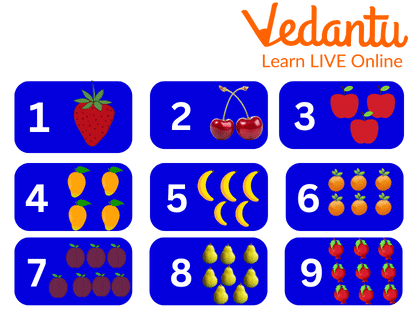Courses
Courses for Kids
Free study material
Offline Centres
MoreLast updated date: 04th Dec 2023
Total views: 101.7k
Views today: 2.01k## Introduction

The range of one-digit numbers is 0 to 9. Since all numbers sum to zero, the total is the original number. When the sum of the digits in the ones position is greater than nine, we regroup a number into tens and ones.

### What are 1 Digit Numbers?

One-digit numbers are from 0 to 9. In the case of whole numbers, 0 is the smallest one-digit number, and in the case of natural numbers, 1 is the smallest one-digit number.Showing Numbers

Having a percentage or number that is nine or less. an increase of one digit. Finding the sum of two or more sets of objects or numbers is what is meant by addition.

### Illustration for Addition of 1 Digit NumbersIllustration for Addition of 1 Digit Numbers

There are 6 stars in row 1.

3 stars are there in row 2.

Thus there will be 9 stars in total if we count them.

Mathematically we can write:

Number of stars in first row $= 6$

Number of stars in second row $= 3$

Total stars in total

$\begin{array}{l} = 6 + 3\\ = 9\end{array}$

There are 9 stars in total.

### Conclusion

Setting the table, getting change at the store, and participating in some games are just a few examples of how adding and subtracting can be beneficial in daily life. Children learn about addition and subtraction to prepare them for learning about multiplication and division in school. One of the fundamental life skills is addition because, without it, one can never understand Maths.

1: How many fruits are in the image below?Ans: There are 6 fruits in the given image.

$3 + 3 = 6$

2: If a boy has 4 pencils, his father gives him 5 more pencils. How many pencils does the boy have?

Ans: Number of pencils boy have $= 4$

Number of pencils his father gave him $= 5$

Total numbers of pencils boy have $= 9{\rm{ As }}\left[ {4 + 5 = 9} \right]$

Total number of pencils boy have in total = 9

3: If a child has 3 colours, his father gives him 4 more colours. How many colours does the boy have ?

Ans: Number of colours boy have $= 3$

Number of colours his father gave him $= 4$

Total numbers of colours boy have $= 7\left[ {3 + 4 = 7} \right]$

Total number of colours child have in total = 9

## FAQs on 1 Digit Addition

1. What does adding a single digit number tell us?

Any number sums to zero, therefore the sum equals the original number. We regroup a number in tens and ones when the total of the digits in the ones place is higher than 9.

2. Change 94 in word (digit to word) ?

Ninety Four

3. Write the largest one digit number.

The largest one digit number is 9.

4. Is the number 0 a single digit?

In the case of whole numbers, 0 is the smallest one-digit number, and in the case of natural numbers, 1 is the smallest one-digit number.

5. What is regrouping?

Making groups of ten when doing Maths operations like addition or subtraction is known as regrouping. This frequently occurs while dealing with double digits. Technically speaking, it also happens whenever you have a response that is greater than 10.